# Equal Area Criterion

At first we have to know about power stability study. Stability study is the procedure for deciding the stability of a system upon some disturbances and this is followed by several switching actions (ON and OFF). In the power system, the behavior of synchronous machine can have some impacts due to these disturbances. The evaluation of this impact in the stability studies are transient stability studies and steady state stability studies. The steady state stability study refers to whether the synchronism is retained or not when the system is subjected to small disturbances. The transient stability studies implies that whether the synchronism is retained or not when the system is subjected to large or severe disturbances.
These disturbances may be a short circuit, application or a loss of a sudden large load or a loss of generation. The objective of this study is to find out whether the load angle comes back to steady value subsequently clearing of the disturbance. Here, non-linear equations are solved to determine the stability. The Equal Area Criterion is concerned with transient stability. It is in fact a very easy graphical method used. It is for deciding the transient stability of single machine or else two-machine system against infinite bus.

## Equal Area Criterion for Stability

Over a lossless line, the real power transmitted will be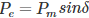Consider a fault occurs in a synchronous machine which was operating in steady state. Here, the power delivered is given by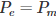For clearing a fault, the circuit breaker in the faulted section should have to be opened up. This process takes 5/6 cycles and the successive post-fault transient will take an additional few cycles.

The prime mover which is giving the input power is driven with the steam turbine. For turbine mass system, the time constant is in the order of few seconds and for the electrical system, it is in milliseconds. Thus, while the electric transients take place, the mechanical power remains stable. The transient study mainly looks into the capability of the power system to retrieve from the fault and to give the stable power with a new probable load angle (δ).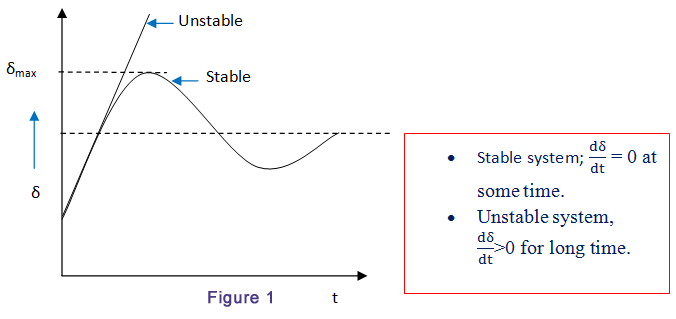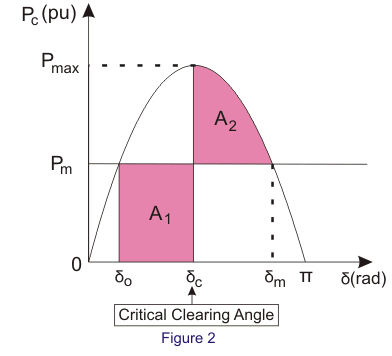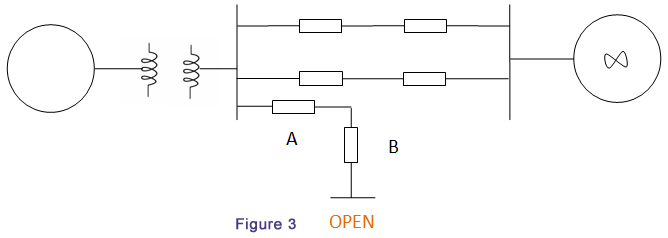The power angle curve is considered which is shown in fig.1. Imagine a system delivering ‘Pm’ power on an angle of δ0 (fig.2) is working in a steady state. When a fault occurs; the circuit breakers opened and the real power is decreased to zero. But the Pm will be stable. As a result, accelerating power,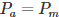The power differences will result in rate of change of kinetic energy stored within the rotor masses. Therefore, due to the stable influence of non-zero accelerating power, the rotor will accelerate. Consequently, the load angle (δ) will increase.
Now, we can consider an angle δc at which the circuit breaker re-closes. The power will then come back to the usual operating curve. At this moment, the electrical power will be higher than the mechanical power. But, the accelerating power (Pa) will be negative. Therefore, the machine will get decelerate. The load power angle will still continue to increase because of the inertia in the rotor masses. This increase in load power angle will stop in due course and rotor of the machine will start to decelerate or else the synchronisation of the system will get lose.

The Swings equation is given by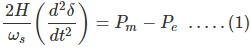Pm → Mechanical power
Pe → Electrical power
H → Inertia constant
ωs → Synchronous speed
We know that,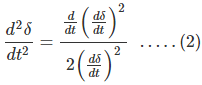Putting equation (2) in equation (1), we get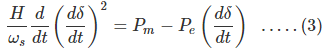Now, multiply dt to either side of equation (3) and integrate it among the two arbitrary load angles which are δ0 and δc. Then we get,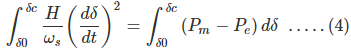Assume the generator is at rest when load angle is δ0. We know that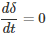At the time of occurrence of a fault, the machine will start to accelerate. When the fault is cleared, it will continue to increase speed before it reaches to its peak value (δc). At this point,So the area of accelerating from equation (4) is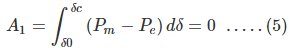Similarly, the area of deceleration is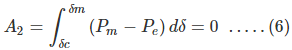Next, we can assume the line to be reclosed at load angle, δc. In this case, the area of acceleration is bigger than area of deceleration. A1 > A2. The load angle of the generator will pass the point δm. Beyond this point, the mechanical power is greater than electrical power and it forces the accelerating power to remain positive. Before slowing down, the generator therefore gets accelerate. Consequently, the system will become unstable.
When A2 > A1, the system will decelerate entirely before getting accelerated again. Here, the rotor inertia will force the successive acceleration and deceleration areas to become smaller than the previous ones. Consequently, the system will reach steady state.
When A2 = A1, the margin of the stability limit is defined by this condition. Here, the clearing angle is given by δcr, the critical clearing angle.
Since, A2 = A1. We get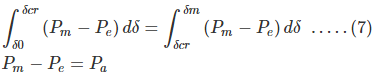The critical clearing angle is related to the equality of areas, it is termed as equal area criterion. It can be used to find out the utmost limit on the load which the system can acquire without crossing the stability limit.

Want To Learn Faster? 🎓
Get electrical articles delivered to your inbox every week.
No credit card required—it’s 100% free.